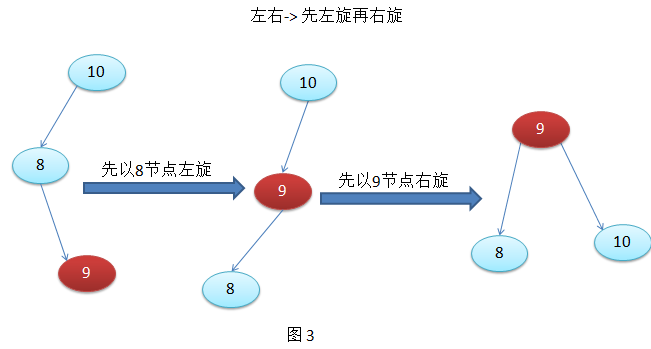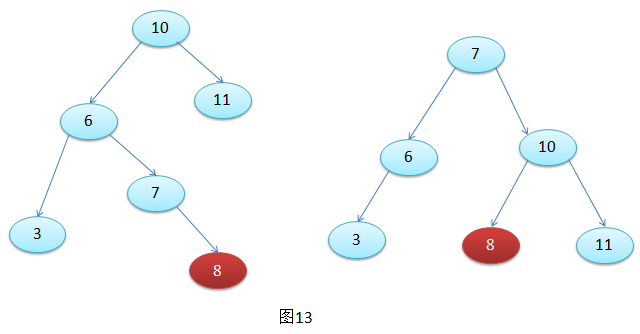# PerKins Zhu

Le vent se lève,il faut tenter de vivre.

### 导航

二叉排序树集中了数组的查找优势以及链表的插入、删除优势，因此在数据结构中占有一定的地位。但在一定的情况下二叉排序树又有可能变为链表，例如插入从1~100的数，这时进行数据查找的效率就要降低。

如何让二叉树的左右子树深度差不超过1呢？这就需要对节点进行旋转，也就是当某个节点的左右子树深度超过1时需要对这个节点进行旋转（旋转之后依旧是左子树小于节点小于右子树），重新调整树的结构。右：节点插入在最小不平衡树的右子树的右子树上面。　　　右左：节点插入在最小不平衡树的右子树的左子树上面。

左左：右旋如果该节点没有左右子树（该节点为叶子节点）或者只有其中一个子树则可以直接进行删除

否则需要继续进行判定该节点：如果该节点的外部（内外：以根节点做对称轴，靠近对称轴的子树为内部子树）子树树高低于内部子树树高，则找到该节点内部子树的最值（最值：如果内部子树是该节点的右子树则数值为右子树的最小值；如果内部节点是该节点的左子树则数值为该节点左子树的最大值）进行数值交换，交换之后删除该节点即可。这就要根据最小不平衡节点的左节点6进行判定，如果6的左节点树高低于6的右节点树高则需要进行一次左旋，最后进行一次右旋结束。

如果6的左子树树高高于6的右子树树高则不需进行左旋可以直接对10节点进行右旋结束操作。

如图15，这种情况肯定需要进行左旋，至于在左旋之前要不要对13节点进行右旋，相信知道该如何判断了。

根据13节点的左右子树高度来判断，左子树（内部）高于右子树（外部）高度则需要进行左旋，图15这种情景是不需要的。package com.zpj.datastructure.avlTree;

/**
* @author PerKins Zhu
* @date:2016年8月30日 下午8:01:03
* @version :1.1
*
*/
// 存储数据类型必须实现Comparable接口，实现比较方法
public class AVLTree<T extends Comparable<T>> {
private Node<T> root;

// 定义节点存储数据
private static class Node<T> {
Node<T> left;// 左孩子
Node<T> right;// 右孩子
T data; // 存储数据
int height; // 树高

public Node(Node<T> left, Node<T> right, T data) {
this.left = left;
this.right = right;
this.data = data;
this.height = 0;
}
}

// 对外公开的方法进行插入
public Node<T> insert(T data) {
return root = insert(data, root);
}

// 私有方法进行递归插入，返回插入节点
private Node<T> insert(T data, Node<T> node) {
// 递归终止条件
if (node == null)
return new Node<T>(null, null, data);
// 比较插入数据和待插入节点的大小
int compareResult = data.compareTo(node.data);
if (compareResult > 0) {// 插入node的右子树
node.right = insert(data, node.right);
// 回调时判断是否平衡
if (getHeight(node.right) - getHeight(node.left) == 2) {// 不平衡进行旋转
// 判断是需要进行两次旋转还是需要进行一次旋转
int compareResult02 = data.compareTo(node.right.data);
if (compareResult02 > 0)// 进行一次左旋(右右)
node = rotateSingleLeft(node);
else
// 进行两次旋转，先右旋，再左旋
node = rotateDoubleLeft(node);
}
} else if (compareResult < 0) {// 插入node的左子树
node.left = insert(data, node.left);
// 回调时进行判断是否平衡
if (getHeight(node.left) - getHeight(node.right) == 2) {// 进行旋转
// 判断是需要进行两次旋转还是需要进行一次旋转
int intcompareResult02 = data.compareTo(node.left.data);
if (intcompareResult02 < 0)// 进行一次左旋(左左)
node = rotateSingleRight(node);
else
// 进行两次旋转，先左旋，再右旋
node = rotateDoubleRight(node);
}
}
// 重新计算该节点的树高
node.height = Math.max(getHeight(node.left), getHeight(node.right)) + 1;
return node;
}

// 右右情况--进行左旋
private Node<T> rotateSingleLeft(Node<T> node) {
Node<T> rightNode = node.right;
node.right = rightNode.left;
rightNode.left = node;
// 旋转结束计算树高
node.height = Math.max(getHeight(node.left), getHeight(node.right)) + 1;
rightNode.height = Math.max(node.height, getHeight(rightNode.right)) + 1;
return rightNode;
}

// 左左情况--进行右旋
private Node<T> rotateSingleRight(Node<T> node) {
Node<T> leftNode = node.left;
node.left = leftNode.right;
leftNode.right = node;
// 旋转结束计算树高
node.height = Math.max(getHeight(node.left), getHeight(node.right)) + 1;
leftNode.height = Math.max(getHeight(leftNode.left), node.height) + 1;
return leftNode;
}

// 右左情况--先右旋再左旋
private Node<T> rotateDoubleLeft(Node<T> node) {
// 先进行右旋
node.right = rotateSingleRight(node.right);
// 再加上左旋
node = rotateSingleLeft(node);
return node;
}

// 左右--先左旋再右旋
private Node<T> rotateDoubleRight(Node<T> node) {
// 先进行左旋
node.left = rotateSingleLeft(node.left);
// 在进行右旋
node = rotateSingleRight(node);
return node;
}

// 计算树高
private int getHeight(Node<T> node) {
return node == null ? -1 : node.height;
}

// public 方法供外部进行删除调用
public Node<T> remove(T data) {
return root = remove(data, root);
}

// 递归进行删除，返回比较节点
private Node<T> remove(T data, Node<T> node) {
if (node == null) {// 不存在此节店，返回null.不需要调整树高
return null;
}
int compareResult = data.compareTo(node.data);
if (compareResult == 0) {// 存在此节点进入
/**
* 找到节点之后进行节点删除操作 判断node是否有子树，如果没有子树或者只有一个子树则直接进行删除
*     如果有两个子树，则需要判断node的平衡系数balance
*         如果balance为0或者1则把node和node的左子树的最大值进行交换 否则把node和右子树的最小值进行交换
*         交换数据之后删除该节点 删除之后判断delete节点的父节点是否平衡，如果不平衡进行节点旋转
* 旋转之后返回delete节点的父节点进行回溯
* */
if (node.left != null && node.right != null) { // 此节点存在左右子树
// 判断node节点的balance，然后进行数据交换删除节点
int balance = getHeight(node.left) - getHeight(node.right);
Node<T> temp = node;// 保存需要进行删除的node节点
if (balance == -1) {
// 与右子树的最小值进行交换
exChangeRightData(node, node.right);
} else {
// 与左子树的最大值进行交换
exChangeLeftData(node, node.left);
}
// 此时已经交换完成并且把节点删除完成，则需要重新计算该节点的树高
temp.height = Math.max(getHeight(temp.left), getHeight(temp.right)) + 1;
// 注意此处，返回的是temp,也就是保存的需要删除的节点，而不是替换的节点
return temp;
} else {
// 把node的子节点返回调用处等于删除了node节点
// 此处隐含了一个node.left ==null && node.right == null 的条件，这时返回null
return node.left != null ? node.left : node.right;
}
} else if (compareResult > 0) {// 没找到需要删除的节点继续递归进行寻找
node.right = remove(data, node.right);
// 删除之后进行树高更新
node.height = Math.max(getHeight(node.left), getHeight(node.right)) + 1;
// 如果不平衡则进行右旋调整。
if (getHeight(node.left) - getHeight(node.right) == 2) {// 进行旋转
Node<T> leftSon = node.left;
// 判断是否需要进行两次右旋还是一次右旋
// 判断条件就是比较leftSon节点的左右子节点树高
if (leftSon.left.height > leftSon.right.height) {
// 右旋一次
node = rotateSingleRight(node);
} else {
// 两次旋转，先左旋，后右旋
node = rotateDoubleRight(node);
}
}
return node;
} else if (compareResult < 0) {// 没找到需要删除的节点继续递归进行寻找
node.left = remove(data, node.left);
// 删除之后进行树高更新
node.height = Math.max(getHeight(node.left), getHeight(node.right)) + 1;
// 如果不平衡进行左旋操作
if (getHeight(node.left) - getHeight(node.right) == 2) {// 进行旋转
Node<T> rightSon = node.right;
// 判断是否需要进行两次右旋还是一次右旋
// 判断条件就是比较rightSon节点的左右子节点树高
if (rightSon.right.height > rightSon.left.height) {
node = rotateSingleLeft(node);
} else {
// 先右旋再左旋
node = rotateDoubleLeft(node);
}
}
return node;
}
return null;
}

// 递归寻找right节点的最大值
private Node<T> exChangeLeftData(Node<T> node, Node<T> right) {
if (right.right != null) {
right.right = exChangeLeftData(node, right.right);
} else {
// 数据进行替换
node.data = right.data;
// 此处已经把替换节点删除
return right.left;
}
right.height = Math.max(getHeight(right.left), getHeight(right.right)) + 1;
// 回溯判断left是否平衡,如果不平衡则进行左旋操作。
int isbanlance = getHeight(right.left) - getHeight(right.right);
if (isbanlance == 2) {// 进行旋转
Node<T> leftSon = node.left;
// 判断是否需要进行两次右旋还是一次右旋
// 判断条件就是比较leftSon节点的左右子节点树高
if (leftSon.left.height > leftSon.right.height) {
// 右旋一次
return node = rotateSingleRight(node);
} else {
// 两次旋转，先左旋，后右旋
return node = rotateDoubleRight(node);
}
}
return right;
}

// 递归寻找left节点的最小值
private Node<T> exChangeRightData(Node<T> node, Node<T> left) {
if (left.left != null) {
left.left = exChangeRightData(node, left.left);
} else {
node.data = left.data;
// 此处已经把替换节点删除
return left.right;
}
left.height = Math.max(getHeight(left.left), getHeight(left.right)) + 1;
// 回溯判断left是否平衡,如果不平衡则进行左旋操作。
int isbanlance = getHeight(left.left) - getHeight(left.right);
if (isbanlance == -2) {// 进行旋转
Node<T> rightSon = node.right;
// 判断是否需要进行两次右旋还是一次右旋
// 判断条件就是比较rightSon节点的左右子节点树高
if (rightSon.right.height > rightSon.left.height) {
return node = rotateSingleLeft(node);
} else {
// 先右旋再左旋
return node = rotateDoubleLeft(node);
}
}
return left;
}

// ************************中序输出  输出结果有小到大*************************************
public void inorderTraverse() {
inorderTraverseData(root);
}

// 递归中序遍历
private void inorderTraverseData(Node<T> node) {
if (node.left != null) {
inorderTraverseData(node.left);
}
System.out.print(node.data + "、");
if (node.right != null) {
inorderTraverseData(node.right);
}
}

}

package com.zpj.datastructure.avlTree;

import org.junit.Test;

/**
* @author PerKins Zhu
* @date:2016年8月30日 下午8:42:15
* @version :1.1
*
*/
public class AVLTreeTest {

@Test
public void test01() {
AVLTree tree = new AVLTree();
int array[] = { 28, 35, 5, 35, 26, 30, 1, 21, 18, 35, 7, 30, 25, 1, 7, };
for (int i = 0; i < array.length; i++) {
System.out.print(array[i] + ",");
tree.insert(array[i]);
}
System.out.println();
tree.inorderTraverse();
tree.remove(12);
System.out.println();
tree.inorderTraverse();
}

@Test
public void test02() {
AVLTree tree = new AVLTree();
int temp = 0;
for (int i = 0; i < 15; i++) {
int num = (int) (Math.random() * 40);
System.out.print(num + ",");
tree.insert(num);
temp = num;
}
System.out.println();
tree.inorderTraverse();
tree.remove(temp);// 删除插入的最后一个数据
System.out.println();
tree.inorderTraverse();
}

}

------------------------------------------------------